# Diagnostic for fine-mapping with summary statistics

#### 2023-02-17

This vignette demonstrates the use of the diagnostic plot for assessing consistency of the summary statistics and the reference LD matrix.

The susie_rss assumes the LD matrix accurately estimate the correlations among SNPs from the original GWAS genotype data. Typically, the LD matrix comes from some public database of genotypes in a suitable reference population. The inaccurate LD information leads to unreliable fine-mapping result.

The diagnostic for consistency between summary statistics and refenrence LD matrix is based on the RSS model under the null with regularized LD matrix. $\hat{z} | R, \lambda \sim N(0, (1-\lambda)R + \lambda I), 0<\lambda<1$ The parameter $$\lambda$$ is estimated by maximum likelihood. A larger $$\lambda$$ means a greater inconsistency between summary statistics and the LD matrix. The expected z score is computed for each SNP, $$E(\hat{z}_j | \hat{z}_{-j})$$, and plotted against the observed z scores.

library(susieR)
library(curl)

## LD information from the original genotype data

We demonstrate the diagnostic plot in a simple case, the LD matrix is estimated from the original genotype data. In this case, we expect the diagnostic plot to confirm that the LD matrix is consistent with the z scores.

We use the same simulated data as in fine mapping vignette.

data("N3finemapping")
n = nrow(N3finemapping$X) b = N3finemapping$true_coef[,1]
sumstats <- univariate_regression(N3finemapping$X, N3finemapping$Y[,1])
z_scores <- sumstats$betahat / sumstats$sebetahat
Rin = cor(N3finemapping$X) attr(Rin, "eigen") = eigen(Rin, symmetric = TRUE) susie_plot(z_scores, y = "z", b=b)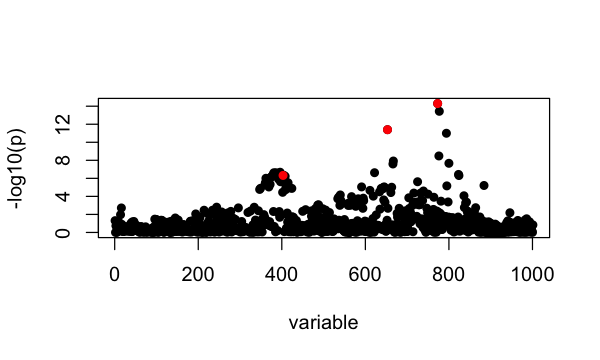The estimated $$\lambda$$ is lambda = estimate_s_rss(z_scores, Rin, n=n) lambda #  6.474096e-09 The plot for the observed z scores vs the expected z scores is condz_in = kriging_rss(z_scores, Rin, n=n) condz_in$plot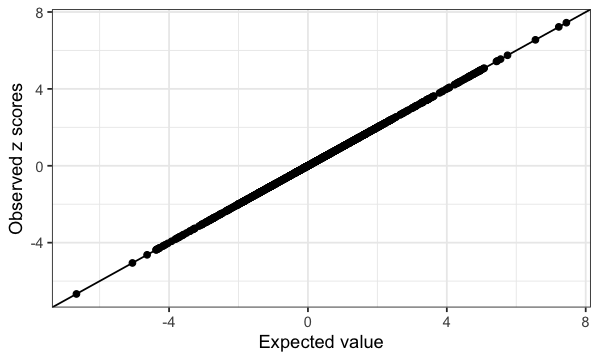Summary of SuSiE Credible Sets:

fit <- susie_rss(z_scores, Rin, n=n, estimate_residual_variance = TRUE)
# HINT: For estimate_residual_variance = TRUE, please check that R is the "in-sample" LD matrix; that is, the correlation matrix obtained using the exact same data matrix X that was used for the other summary statistics. Also note, when covariates are included in the univariate regressions that produced the summary statistics, also consider removing these effects from X before computing R.
susie_plot(fit,y = "PIP", b=b)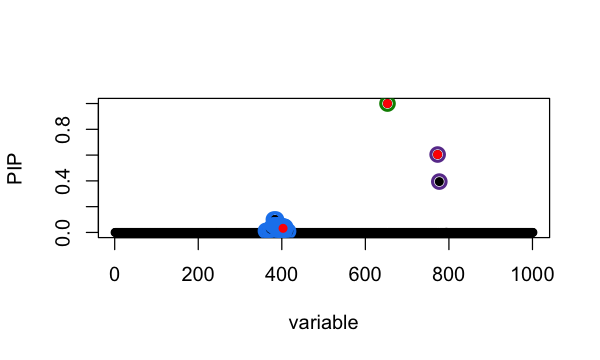## LD information from the reference panel

We use another simulated data where the LD matrix is estimated from a reference panel. In this example data set, there is one association signal in the simulated data (red point), and there is one SNP with mismatched reference and alternative allele between summary statistics and the reference panel (yellow point).

Note: In some versions of PLINK, these mismatches can occur when PLINK automatically flips the alleles to make the minor allele be the effect allele, leading to different allele encodings in the z scores and LD matrix. Adding the flag --keep-allele-order will disable this behaviour in PLINK.

data_file <- tempfile(fileext = ".RData")
data_url <- paste0("https://raw.githubusercontent.com/stephenslab/susieR/",
"master/inst/datafiles/SummaryConsistency1k.RData")
zflip = SummaryConsistency$z ld = SummaryConsistency$ldref
n=10000
b = numeric(length(zflip))
b[SummaryConsistency$signal_id] = zflip[SummaryConsistency$signal_id]
plot(zflip, pch = 16, col = "#767676", main = "Marginal Associations",
xlab="SNP", ylab = "z scores")
points(SummaryConsistency$signal_id, zflip[SummaryConsistency$signal_id], col=2, pch=16)
points(SummaryConsistency$flip_id, zflip[SummaryConsistency$flip_id], col=7, pch=16)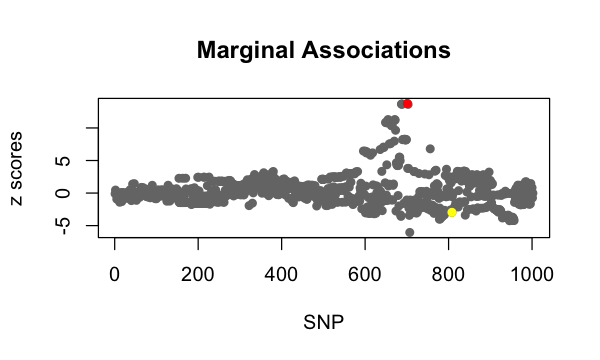Using the data with misaligned allele, SuSiE-RSS identifies a true positive CS containing the true effect SNP; and a false positive CS that incorrectly contains the mismatched SNP.

fit = susie_rss(zflip, ld, n=n)
susie_plot(fit, y='PIP', b=b)
points(SummaryConsistency$flip_id, fit$pip[SummaryConsistency$flip_id], col=7, pch=16)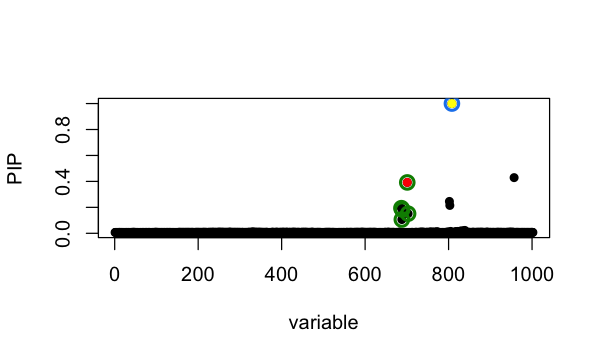The estimated $$\lambda$$ is lambda = estimate_s_rss(zflip, ld, n=n) lambda #  0.005005011 In the diagnostic plot, the mismatched SNP shows the largest difference between observed and expected z-scores, and therefore appears furthest away from the diagonal. condz = kriging_rss(zflip, ld, n=n) condz$plot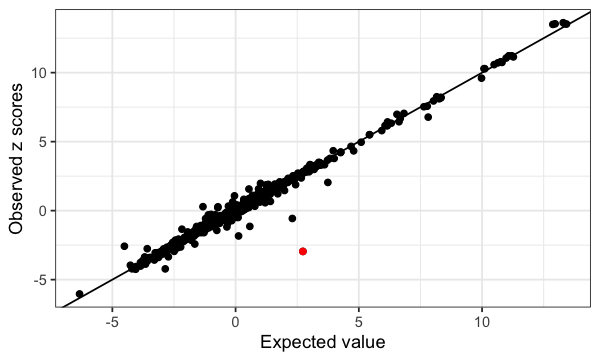After fixing the allele encoding, SuSiE-RSS identifies a single true positive CS containing the true-effect SNP, and the formerly mismatched SNP is (correctly) not included in a CS.

z = zflip
z[SummaryConsistency$flip_id] = -z[SummaryConsistency$flip_id]
susie_plot(fit, y='PIP', b=b)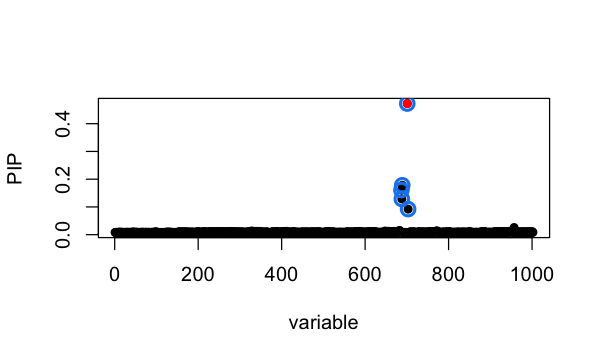## Session information

Here are some details about the computing environment, including the versions of R, and the R packages, used to generate these results.

sessionInfo()
# R version 3.6.2 (2019-12-12)
# Platform: x86_64-apple-darwin15.6.0 (64-bit)
# Running under: macOS Catalina 10.15.7
#
# Matrix products: default
# BLAS:   /Library/Frameworks/R.framework/Versions/3.6/Resources/lib/libRblas.0.dylib
# LAPACK: /Library/Frameworks/R.framework/Versions/3.6/Resources/lib/libRlapack.dylib
#
# locale:
#  en_US.UTF-8/en_US.UTF-8/en_US.UTF-8/C/en_US.UTF-8/en_US.UTF-8
#
# attached base packages:
#  stats     graphics  grDevices utils     datasets  methods   base
#
# other attached packages:
#  curl_4.3       Matrix_1.2-18  susieR_0.12.35
#
# loaded via a namespace (and not attached):
#   tidyselect_1.1.1   xfun_0.29          bslib_0.3.1        purrr_0.3.4
#   lattice_0.20-38    colorspace_1.4-1   vctrs_0.3.8        generics_0.0.2
#   htmltools_0.5.2    yaml_2.2.0         utf8_1.1.4         rlang_1.0.6
#  mixsqp_0.3-46      jquerylib_0.1.4    pillar_1.6.2       glue_1.4.2
#  DBI_1.1.0          RcppZiggurat_0.1.5 matrixStats_0.63.0 lifecycle_1.0.0
#  plyr_1.8.5         stringr_1.4.0      munsell_0.5.0      gtable_0.3.0
#  evaluate_0.14      labeling_0.3       knitr_1.37         fastmap_1.1.0
#  parallel_3.6.2     irlba_2.3.3        fansi_0.4.0        Rfast_2.0.3
#  highr_0.8          Rcpp_1.0.8         scales_1.1.0       jsonlite_1.7.2
#  farver_2.0.1       ggplot2_3.3.6      digest_0.6.23      stringi_1.4.3
#  dplyr_1.0.7        grid_3.6.2         cli_3.5.0          tools_3.6.2
#  magrittr_2.0.1     sass_0.4.0         tibble_3.1.3       crayon_1.4.1
#  pkgconfig_2.0.3    ellipsis_0.3.2     assertthat_0.2.1   rmarkdown_2.11
#  reshape_0.8.8      R6_2.4.1           compiler_3.6.2